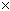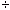Question: How do I find out the square footage of a window 45" by 22" and 43" by 35.5"? Hi there. When you have length measurements in inches and want to find area in square feet, you first find the area in square inches and then convert that to square feet. In square inches then, a 45" by 22" window would be 4522 = 990 square inches. There are twelve inches in a foot, but since we have two dimensions we are converting from inches to feet, we divide by twelve twice: 9901212 = 6 7 /8 square feet. Stephen La Rocque. >